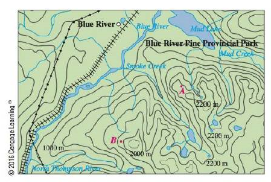Chapter 14.6, Problem 36E

Chapter
Section
Textbook Problem

Shown is a topographic map of Blue River Pine Provincial Pork in British Columbia. Draw curves of steepest descent from point A (descending to Mud Lake) and from point B.To determine

To draw: The curves of steepest descent from point A and from point B .

Explanation

Start with point A and sketch the curves which is perpendicular to the contour curves and move towards Mud Lake.

Start with point B and sketch the curves which is perpendicular to the contour curves and move towards Mud Lake

Still sussing out bartleby?

Check out a sample textbook solution.

See a sample solution

The Solution to Your Study Problems

Bartleby provides explanations to thousands of textbook problems written by our experts, many with advanced degrees!

Get Started

Sketch the graphs of the equations in Exercises 512. x2y=1

Finite Mathematics and Applied Calculus (MindTap Course List)

Find the derivative of the function. F(x)=x1t+sintdt

Single Variable Calculus: Early Transcendentals, Volume I

Draw a polygon for the distribution of scores shown in the following table. X f 6 2 5 5 4 3 3 2 2 1

Essentials of Statistics for The Behavioral Sciences (MindTap Course List)

In Exercises 4148, find the indicated limit given that limxaf(x)=3 and limxag(x)=4 41. limxa[f(x)g(x)]

Applied Calculus for the Managerial, Life, and Social Sciences: A Brief Approach

In Problems 33 – 38, solve each inequality. 33.

Mathematical Applications for the Management, Life, and Social Sciences

Solve each equation: 3x+14=29

Elementary Technical Mathematics

Finding a Limit In Exercises 47-62, find the limit. limx3x+12x3

Calculus: Early Transcendental Functions (MindTap Course List)

Given: m1=8(x+2)m3=5x3m5=5(x+1)2 Find: x

Elementary Geometry for College Students